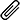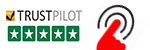High Quality, Fast Delivery, Plagiarism Free - Just in 3 Steps

## Upload Questions Details and Instructions:Select Assignment Files

Assignment solution along with originality report.

Get assignment answer help by skilled & qualified tutors.

### Best Price Guarantee

Friendly pricing & refund policy.# Statistic :Survival Analysis Assignment Help

Stat 4100/5100 – Survival Analysis – Fall 2015
Assignment 8 (Due Wed, Nov 25):
1. Consider a censored sample from the exponential distribution with rate parameter  and xed cen-
soring time c, common to all individuals that were in the study. That is, individual i is censored if
failure time, Xi > c. Otherwise Xi is observed.
(a) Suppose that only the number of failures, D, is observed. Show that D has a binomial distribu-
tion with parameters n and p = 1 exp[c]. Using the relationship between the variance of
maximum likelihood estimators and expected information obtain an expression for the variance
of the maximum likelihood estimate of . This should be a true variance not a standard error;
i.e. an expression that depends upon the parameters of the model, not data.
(b) Suppose now the usual case: (
P
i ti;D) are observed, where the ti are the failure and censoring
times. You showed on Assignment 4 that l00() = D=2 and ^
= D=
P
i ti. Using the relation-
ship between the variance of maximum likelihood estimators and expected information obtain
an expression for the variance of the maximum likelihood estimate of . Again, this should
depend on the parameters of the model, not data.
(c) The eciency of an estimator ^
1 to another estimator ^
2 is the ratio of the large sample variances
of the two estimators: Var(^
2)=Var(^
1). Obtain an expression for the eciency of the estimator
in (a) to the estimator in (b). Note that the eciency depends upon  and c. In R, produce a
plot of the eciency as a function of  for c = 1. For what values of  does the method in (a)
give almost as good results as method (b), if any?
2. 8.6. The data is available on the web site. Be sure to use factor to ensure variables that are
supposed to have interpretations as categorical variables do have such interpretations. In (a), by
ANOVA table, the question means the output of summary in R. In (c) you can answer the question
by nding a 95% con dence interval for the relevant relative risk and checking whether 1 falls in the
interval.#### Get This Answer for Study Help

If you need study assistance with writing your questions and answers, our professional assignment writing service is here to help!

#### Content Removal Request

If you are the original writer or copyright-authorized owner of this article and no longer wish to have, your work published on casestudyhelp.com, then please Request for removal of this content.

Top